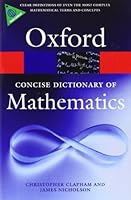## My Wishlist

•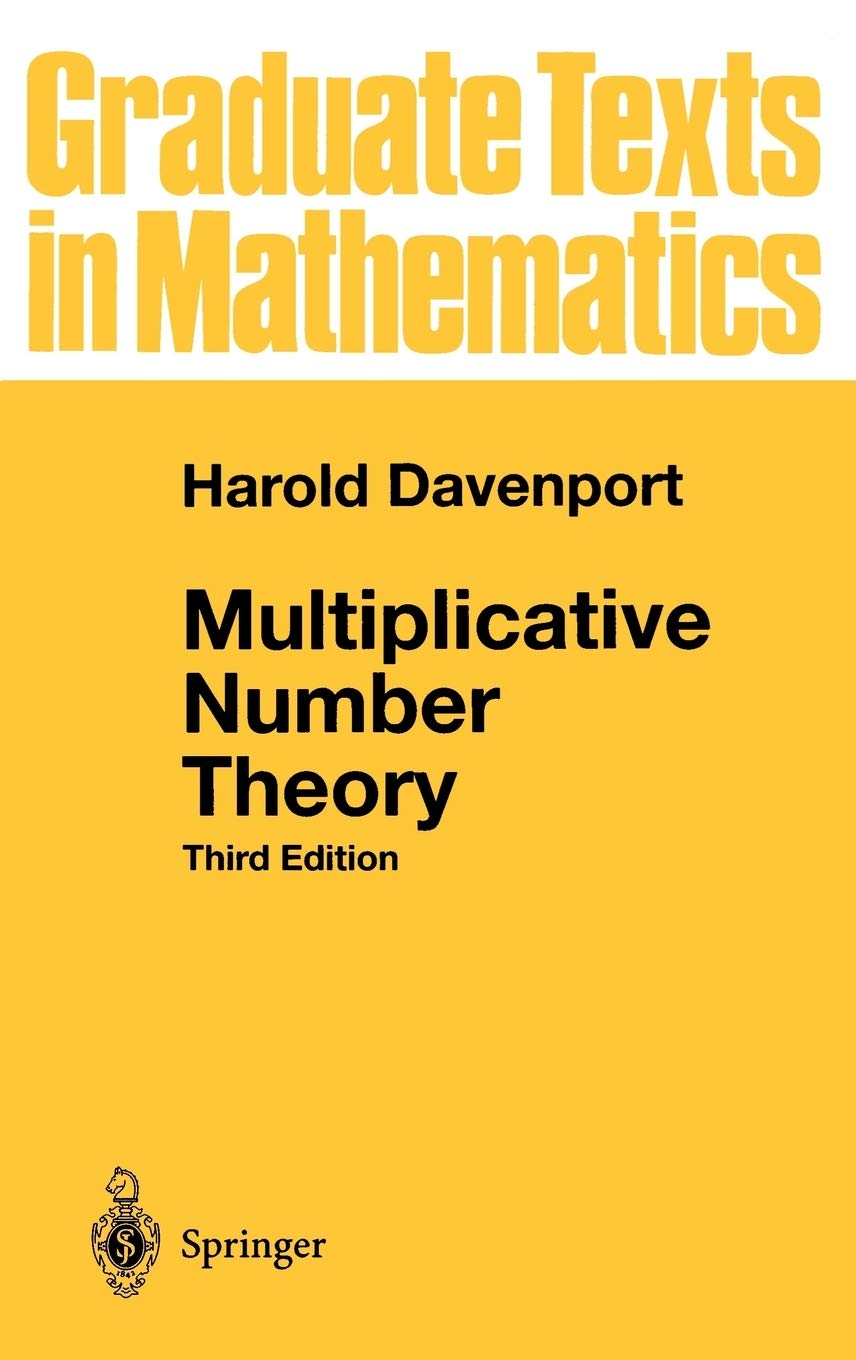#### Multiplicative Number Theory

Davenport is a classical book which I have borrowed from the library over and over again, and which I would like to own.

•#### A Hardback Copy of Homotopy Type Theory

A book offering a foundation of mathematics using types, making things more natural for Computer Science to develop (in particular for functional programming), as opposed to ZF(C).

•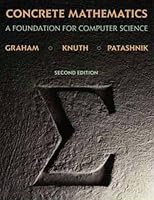#### Concrete Mathematics: Foundation for Computer Science

This book (another classic by Donald Knuth) introduces the mathematics that supports advanced computer programming and the analysis of algorithms.

•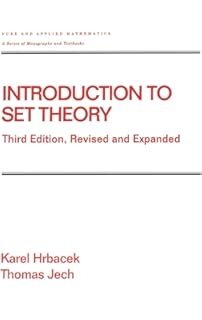#### Introduction to Set Theory

An excellent text on foundations of mathematics and ZF(C).

•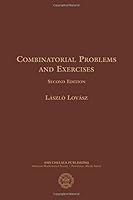#### Combinatorial Problems and Exercises

A book of interesting combinatorial problems by the renowned mathematician László Lovász.

•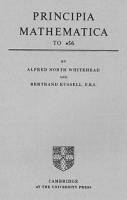#### Principia Mathematica: Volumes 1-3

Not to be confused with Isaac Newton's "Principia", this is an attempt by the mathematical logicians Bertrand Russell and Alfred Whitehead to consistently axiomatise arithmetic.

•#### Algebra: Chapter 0

A nice introduction to algebra which unusually incorporates category theory early on.

•#### Lambda Calculus with Types

A book on lambda calculus and type theory: very interesting.

•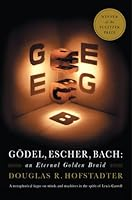#### Godel, Escher, Bach: An Eternal Golden Braid

By exploring common themes in the lives and works of logician Kurt Gödel, artist M. C. Escher and composer Johann Sebastian Bach, the book expounds concepts fundamental to mathematics, symmetry, and intelligence.

•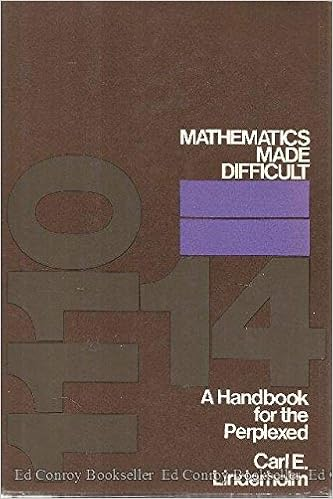A hilarious book which aims to confuse its readers. Includes exercises such as "Show that 17 × 17 = 289. Generalise this result."

•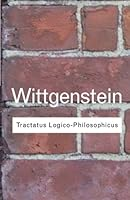#### Tractatus Logico-Philoso​phicus

Ludwig Wittgenstein's only published work, a very influential 20th century work.

•